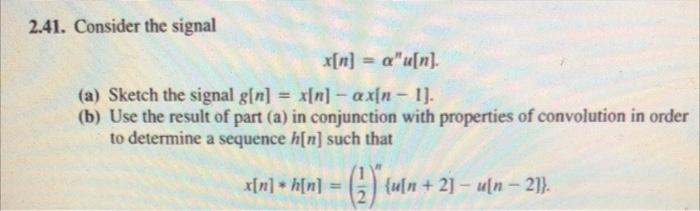# Question 2.41. Consider the signal $x[n]=\alpha^{n} u[n] \text {. }$ (a) Sketch the signal $$g[n]=x[n]-\alpha x[n-1]$$. (b) Use the result of part (a) in conjunction with properties of convolution in order to determine a sequence $$h[n]$$ such that $x[n] * h[n]=\left(\frac{1}{2}\right)^{n}\{u[n+2]-u[n-2]\} .$F8JTDJ The Asker · Electrical EngineeringTranscribed Image Text: 2.41. Consider the signal $x[n]=\alpha^{n} u[n] \text {. }$ (a) Sketch the signal $$g[n]=x[n]-\alpha x[n-1]$$. (b) Use the result of part (a) in conjunction with properties of convolution in order to determine a sequence $$h[n]$$ such that $x[n] * h[n]=\left(\frac{1}{2}\right)^{n}\{u[n+2]-u[n-2]\} .$
More
Transcribed Image Text: 2.41. Consider the signal $x[n]=\alpha^{n} u[n] \text {. }$ (a) Sketch the signal $$g[n]=x[n]-\alpha x[n-1]$$. (b) Use the result of part (a) in conjunction with properties of convolution in order to determine a sequence $$h[n]$$ such that $x[n] * h[n]=\left(\frac{1}{2}\right)^{n}\{u[n+2]-u[n-2]\} .$